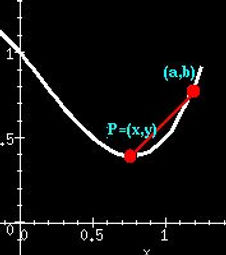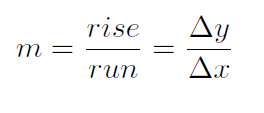# 26th January, 2021

Abstract

This article mainly determines the two bases of calculus namely, differential calculus and integral calculus from the geometric background. Differentiation is performed by calculating the slope of a tangent line drawn graphically to a function. Definite integration of a function, on the other hand, can be measured by calculating the area under the graph of a function by comparing this area to a hypersolid's content using Cavalieri's principle.

1.  The Derivation of Differentiation using Geometry

The instantaneous rate of change of a function with respect to one of its variables is known as a derivative. The derivative of the function can also be obtained by finding the slope of the tangent line to the function at a point. So, in this article, we will view the use of derivatives as tangents to obtain a geometric definition of the derivative.Here, we have to find the slope of the tangent line to a graph at the point P. We can now calculate the slope of any arbitrary function by drawing a line through the point P and another point nearby (in the figure, we take Q with coordinates (a,b)), and then finding the slope of that line which is called the secant line; which can be determined using the following formula:Here, m represents the slope.

Let, P = (x,y) and Q = (a,b). Let us now Assume the values of a & b asThen the slope of the lineNow, we need to decrease the size of Δx as much as possible. We do this by taking the limit as Δx approaches zero. In the limit, assuming the limit exists, we will calculate the exact slope of the tangent line to the curve at the given point, PQ. The value of the derivative, df/dx is given byThis value of the derivative can also be expressed in different ways.  Generally, h is often used in place of Δx. Or Δy is used in place ofThus there are three different ways of expressing the definition of the derivative:1. Derivation of Integration using Geometry

Cavalieri's Principle

Bonaventura Francesco Cavalieri (1598-1647) was a disciple of Galileo. The following portion, known as Cavalieri's principle, is taken from his Book, “Geometria Indivisibilibus Continuorum Nova quadam ratione promota”, Part VII, Theorem 1, Proposition 1:-

“If  between the same parallels any two plane figures are constructed, and if in them, any straight lines being drawn equidistant from the parallels, the included portions of any one of these lines are equal, the plane figures are also equal to one another, and if between the same parallel planes any solid figures are constructed, and if in them, any planes being drawn equidistant from the parallel planes, the included plane figures out of any one of the planes so drawn are equal, the solid figures are likewise equal to one another.”

As an application of Cavalieri's principle, let us now find a connection between the volumes of a cylinder, a cone, and a sphere.Let us now assume that the height of a cylinder is equal to the radius of its base r and that a cone with the same base and height is inscribed in the cylinder, as shown above.

Measuring from the base, the area of the cross-section of the cone at level y equals πy². The cross-section of the cylinder which is independent of its height is equal to πr², which means that the area of the remaining disc (at height y) is equal to π(r² - y²). By using the Pythagorean Theorem, this is equal to the area of the cross-section of the hemisphere of radius r at the same value of height y. By using Cavalieri's principle, we observe that the volume of the cylinder is less than the volume of the cone equals the volume of the hemisphere.

We know that the volume of a cylinder is equal to the product of cross-section and the height, the volume of the cone is 1/3 of that. Therefore, the volume of the hemisphere is equal to 2/3 of the volume of the cylinder, and the volume of the whole sphere is equal to 4/3 of the volume of the cylinder. The latter is πr³, making the volume of the sphere 4/3πr³.

Thus, Cavalieri’s Principle proves to be a simple corollary of a basic integral formula for the volume of a 3-dimensional solid S in terms of the areas of the planar sections St which is formed by intersecting S with the horizontal planes z = t, where t runs through all real numbers which are the third coordinate of all the points in S.

References

1. D. E. Smith, A Source Book in Mathematics, Dover, 1959

2. D. J. Struik, A Source Book in Mathematics 1200-1800, Princeton University Press, 1990 (Third printing)